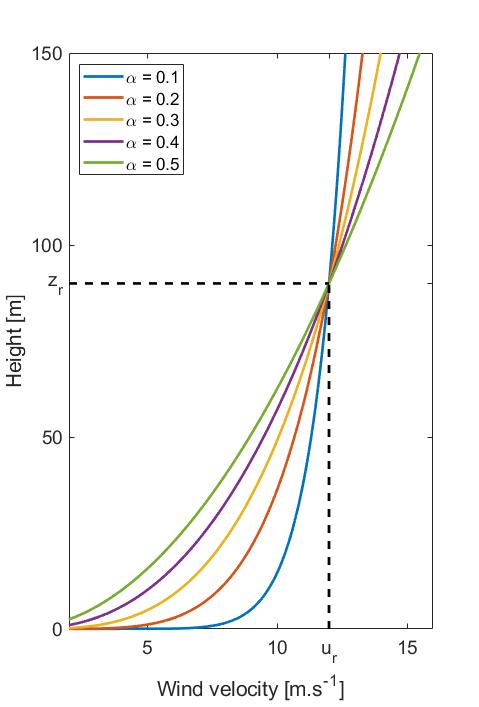# Wind profile power law

In order to estimate the vertical variation of the wind speed
$$u(z)$$
, it is common to use a Power law as defined by the following equation (see for example Hansen (2008c)):

$$u(z) = u_r \cdot \left( z \over z_r \right)^\alpha$$

where
• $$z$$
is the vertical coordinate, typically equal to 0 at the ground or at the MSL - Mean Sea Level
• $$u_r$$
is a reference wind speed, generally obtained from measurements at a reference hight. This is the wind speed that is entered as Initial wind speed for example in Uniform wind or Turbulent wind.
• $$z_r$$
is the reference height, often taken as the hub height (and that can be changed with the Reference height parameter in Uniform wind)
• $$\alpha$$
is the power law exponent (that can be changed with the Power law exponent parameter in Uniform wind)
The image below shows different vertical variations of the wind speed for different power law exponents, for a reference wind speed of 12 m.s-1 and a reference height of 90 m.The default value for the powre law exponent in Ashes is 0.2. Hansen (2008c) recommends values between 0.1 and 0.25.

As can be seen from the equation, a value of 0 will give a uniform vertical distribution (i.e. no variation) and a value of 1 will give a linear variation